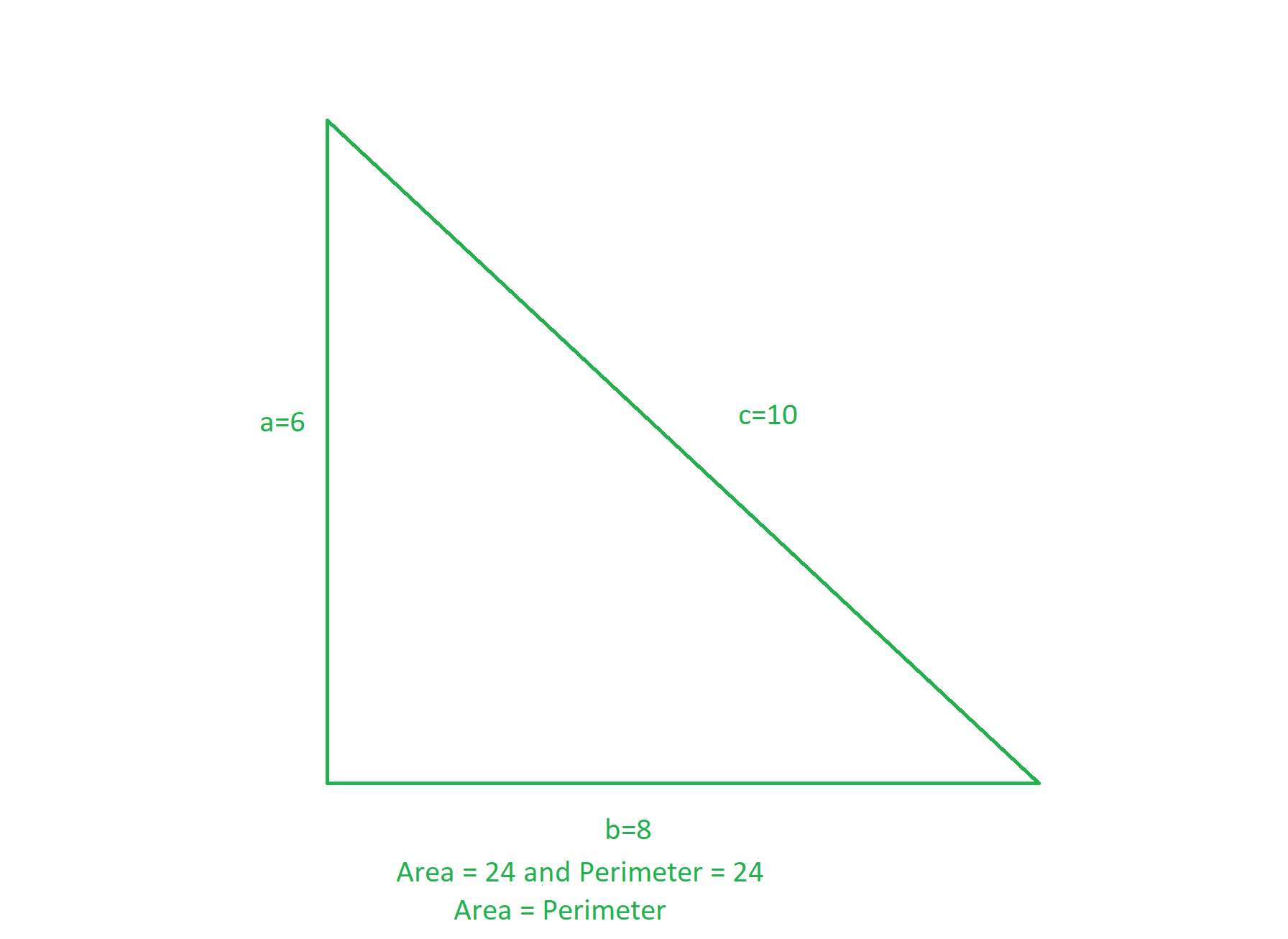Related Articles
Program to find all possible triangles having same Area and Perimeter
• Difficulty Level : Easy
• Last Updated : 16 Dec, 2020

The task is to find all possible triangles having the same perimeter and area.

Examples:The triangle having sides (6, 8, 10) have the same perimeter (= (6 + 8 + 10) = 24) and area (= 0.5 * 6 * 8 = 24).

Approach: The idea is based on the observation from Heron’s Formula. Below are the observations:

Let the sides of the triangle be a, b, c.
Perimeter(P) = a + b + c
Area(A) using Heron’s Formula:where s = (a + b + c) / 2

Experimental Observation:

We know that:
4 * s2 = s * (s – a) * (s – b) * (s – c)
=> 4 * s = (s – a) * (s – b) * (s – c)
=> 2 * 2 * 2 * 4 * s = 2 * (s – a) * 2 * (s -b) * 2 * (s – c)
=> 16 * (a + b + c) = (- a + b + c) * (a – b + c) * (a + b – c)

Due to this condition:
Max value of (- a + b + c), (a – b + c), (a + b – c) is as follows:
(- a + b + c) * (a – b + c) * (a + b – c) ≤ 16 * 16 * 16
=> 16 * (a + b + c) ≤ 16 * 16 * 16
=> (a + b + c) ≤ 256

From the above equation, the sum of sides of the triangle doesn’t exceed 256 whose perimeter of triangle and area of the triangle can be the same. Therefore, the idea is to iterate three nested loops over the range [1, 256] and print those triplets of sides having the same area and perimeter.

Below is the implementation of the above approach:

## C++

 // C++ program for the above approach   #include  using namespace std;   // Function to print sides of all the // triangles having same perimeter & area void samePerimeterAndArea() {     // Stores unique sides of triangles     set > se;       // i + j + k values cannot exceed 256     for (int i = 1; i <= 256; ++i) {           for (int j = 1; j <= 256; ++j) {               for (int k = 1; k <= 256; ++k) {                   // Find the value of 2 * s                 int peri = i + j + k;                   // Find the value of                 // 2 * ( s - a )                 int mul1 = -i + j + k;                   // Find the value of                 // 2 * ( s - b )                 int mul2 = i - j + k;                   // Find the value of                 // 2 * ( s - c )                 int mul3 = i + j - k;                   // If triplets have same                 // area and perimeter                 if (16 * peri == mul1 * mul2 * mul3) {                       // Store sides of triangle                     vector<int> v = { i, j, k };                       // Sort the triplets                     sort(v.begin(), v.end());                       // Inserting in set to                     // avoid duplicate sides                     se.insert(v);                 }             }         }     }       // Print sides of all desired triangles     for (auto it : se) {         cout << it << " "              << it << " "              << it << endl;     } }   // Driver Code int main() {     // Function call     samePerimeterAndArea();       return 0; }

## Python3

 # Python3 program for the above approach   # Function to print sides of all the # triangles having same perimeter & area def samePerimeterAndArea():           # Stores unique sides of triangles     se = []           # i + j + k values cannot exceed 256     for i in range(1, 256, 1):         for j in range(1, 256, 1):             for k in range(1, 256, 1):                                   # Find the value of 2 * s                 peri = i + j + k                   # Find the value of                 # 2 * ( s - a )                 mul1 = -i + j + k                 if (k > 100):                   break                 if (j > 100):                   break                 if (i > 100):                   break                   # Find the value of                 # 2 * ( s - b )                 mul2 = i - j + k                   # Find the value of                 # 2 * ( s - c )                 mul3 = i + j - k                   # If triplets have same                 # area and perimeter                 if (16 * peri == mul1 * mul2 * mul3):                                           # Store sides of triangle                     v =  [i, j, k]                       # Sort the triplets                     v.sort(reverse = False)                       # Inserting in set to                     # avoid duplicate sides                     se.append(v)                     se.sort(reverse = False)       # Print sides of all desired triangles     temp = []     temp.append(se)     temp.append(se)     temp.append(se)     temp.append(se)     temp.append(se)     for it in temp:         print(it, it, it)   # Driver Code if __name__ == '__main__':           # Function call     samePerimeterAndArea()       # This code is contributed by ipg2016107

Output:

5 12 13
6 8 10
6 25 29
7 15 20
9 10 17

Time Complexity: O(2563)
Auxiliary Space: O(2563)

Attention reader! Don’t stop learning now. Get hold of all the important DSA concepts with the DSA Self Paced Course at a student-friendly price and become industry ready.

My Personal Notes arrow_drop_up
Recommended Articles
Page :